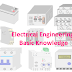In this article, we are going to accrue the knowledge of the basics of electrical engineering. Knowing the electrical engineering basics, you can understand any complex topic of electrical engineering.

The basic knowledge of electrical engineering you must have are the following,

1. You must know what is electricity
2. Important basics terms
3. Electrical Energy Conversion

## Knowledge of Electricity

Electricity is nothing but a source of energy that does so many useful works. The flow of electric power and its effects is also known as electricity. Electricity is a secondary energy source means it can not be found naturally, it extracts from the other source of energy. So electrical engineering mainly deals with electricity to use it for different applications.

## Basics terms of Electrical Engineering

The basic terms and topics you must know before exploring the field of electrical engineering are,

• Electric Current
• Electric Voltage
• Electrical Power
• Resistance
• AC and DC
• Electric Energy Conversion

### Electric Current

In simple words, the flow of electrons or charges through a conductor is called electric current. Every object on this planet made of electrons. When we apply an electric pressure or voltage across them the electrons start moves which are known as electric current. The flow of electric current depends upon the nature of the object(it should be conductor), applied voltage, resistance, size, etc.

### Electric Voltage

The potential difference across a conductor which causes to flow of the electric current through it is called Electric Voltage. It also is known as electric pressure because it forces the electrons to move. Generally, the electrical power sources are the source of electric voltage means they can create a potential difference across the load due to the electric current flow through the load and helps to do the useful work.

### Electrical Power

The rate of electrical energy transferred by an electrical circuit from the power source to load per unit time is called electric power. Also, we can say electrical power is the multiplication of voltage and current. The formula of electric power for AC circuits includes the power factor also. The unit of electric power is the watt. The power consumption depends upon the flow of current and the resistance of the circuit and load.

### Resistance

Resistance is a very important term in electrical as well as electronics engineering. There are so many components used in the electrical circuit but the resistor is a very important and widely used component. Resistor only not having the resistance property, in fact, all the electrical circuits and their components have resistance property.

Resistance is a property of an object or material by virtue of which it opposes the flow of electric current. Resistance shows the same effect for both AC and DC. Resistance causes the production of heat, copper loss, etc.

### AC and DC

According to nature or characteristics, electric power supply divided into two types - 1. Alternating Current or AC 2. Direct Current or DC
In the AC power supply, the magnitude and direction of the current and voltage change with time.
In the DC power supply, the magnitude of the voltage and current is constant, it does not change with time.

The examples of AC power supply source are - alternator, oscillator circuits, inverter circuits

Examples of DC power supply sources are - battery, solar panels, etc.

## Basics of Electrical Energy Conversion

As we know that electricity is the secondary energy source, which means it can get directly from nature. We have to extract the electrical energy from the other source of energy such as,

Mechanical energy to electrical energy - Generator

Chemical energy to electrical energy - Battery

Solar Energy to Electrical Energy - Solar Panels

Also, we cannot use electricity directly. Electrical energy is used by converting it into another form of energy.
For example,

Electric heater converts the electrical energy into heat energy.

Electric Motor converts electrical energy into mechanical energy.

Electric lamps, bulbs converts electrical energy into light energy.

Electrical Engineering Basic Knowledge for AllReviewed by Author on May 21, 2021 Rating: 5